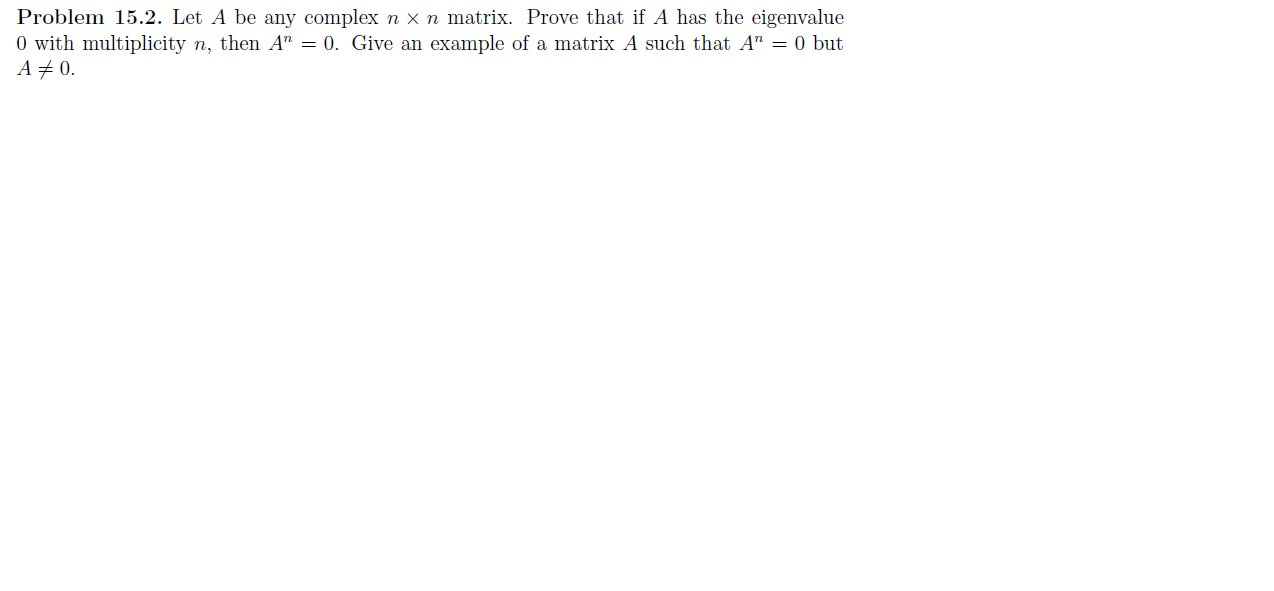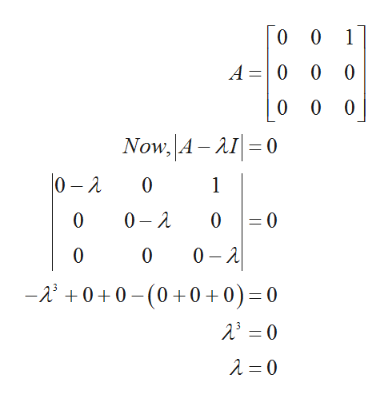# Problem 15.2. Let A be any complex n X n matrix. Prove that if A has the eigenvalue0 with multiplicity n, then A" = 0. Give an example of a matrix A such that A" = 0 butA 0

Question
166 viewshelp_outlineImage TranscriptioncloseProblem 15.2. Let A be any complex n X n matrix. Prove that if A has the eigenvalue 0 with multiplicity n, then A" = 0. Give an example of a matrix A such that A" = 0 but A 0 fullscreen
check_circle

Step 1

Let 3x3 matrix A is given by,

Step 2

Let the Eigen value of the matrix A is λ.

The Eige...help_outlineImage Transcriptionclose0 0 1 A 0 0 0 0 0 0 Now, A-AI0 0-2 0 2 0 1 =0 0 0 0 A 0 0 -0+0(0+0+0) = 0 fullscreen

### Want to see the full answer?

See Solution

#### Want to see this answer and more?

Solutions are written by subject experts who are available 24/7. Questions are typically answered within 1 hour.*

See Solution
*Response times may vary by subject and question.
Tagged in

### Math